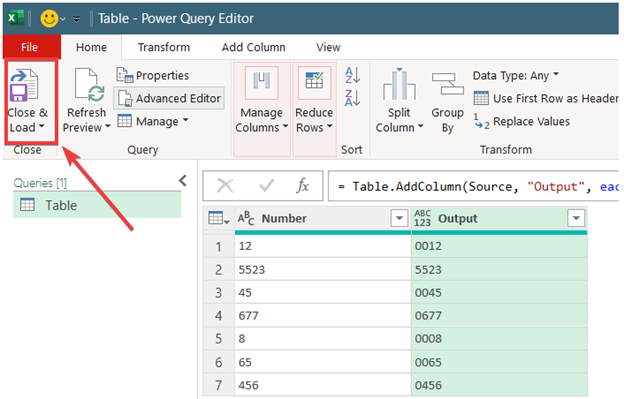# Excel: How to put 0 in front of numbers

Two options are always available for you whenever you want to format text in excel. You can either do it via the Excel format cells option or by using the excel text formula.

You can convert a number into text or vice versa by adding special format strings. Adding zero in front of the text converts the text into a numerical string.

## How to put 0 in front of numbers use the Text Function

Below is the formula for adding zero in front of the text,

"= Text (value, format text)"

For instance, if the cell, A1 contains 1234, and you apply the function, =TEXT(A1, "00000"), the function will display "01234" as a result.

Take a look at =TEXT(A1, "00000")

The function will use the text format in formatting the values inside cell A1. There are five zeroes in the brackets, indicating that the result should have five values.

Now let's consider an example:

Let's consider a table containing STD numbers in the first column named 'A.' We will add 0 to the front of every number on the list.Locate cell B2, select it and enter the formula; =TEXT(A2, "00000000")

Apply the formula by hitting the enter key

Immediately, the numbers will display with zero on the front of the number.Press 'CTRL+C' to copy the formula. Paste it into B3: B10. Alternatively, You can right-click and drag the green rectangle to the rest of the cells.## How to add zero in front of numbers by using the “Format cells option.”

You can add zero in front of each number, as we mentioned earlier. Follow the steps below:

1. First, locate the range of the mobile numbers and select.2. Press the keys "CTRL+1" for the "Format cells" dialog box to pop up3. Check the "number tab" and select "custom"4. You see a box named "Type". Write "0" eight times.

5. Click OkZeros are now in front of each of the numbers.## Using the Apostrophe (') at the Start

Type Apostrophe in front of the zero,## How to add zero in front of all the data that contain both numbers and texts.

We will achieve this by combining IF & ISNUMBER functions.1. Enter the formula, =IF(ISNUMBER(A2)=TRUE,"0"&A2,A2) in cell B2.2. Right-click and Drag the green rectangle downwards, and all the values will display with zero added in front.## Utilizing the Ampersand (&) Symbol

You can use the Ampersand (&) symbol in Excel to add extra zeros in front of numbers. You can also use the trick when adding values of two cells, though in text format. Follow these steps when adding leading 0’s to numbers:

1. Type =“0”&B5 in cell C5.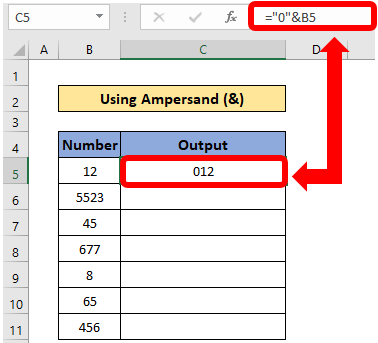2. This will add a zero in front of numbers in cell B5.

3. Use the Fill Handle button to copy the formula into other cells. You can also copy and paste the formula manually into the rest of the cells.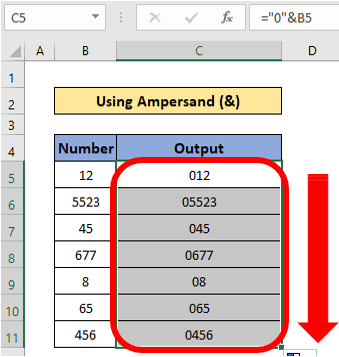4. Note the method will add a zero to all cells; hence, it will not make numbers of the same digits.

## Utilizing Other Excel Functions

Excel also has numerous functions that can add zeros in front of numbers easily. These functions include:

### The REPT Function

The REPT function repeats a character or text a specified number of times. Its syntax is =REPT (text, number_times), where;

text represents the character or text you need to repeat.

number_times is the number of times you want to repeat the text or character.

With this formula, you can proceed with these steps:

1. Write the formula in cell C5, =REPT(0,1)&B52. Copy and paste the formula into other cells.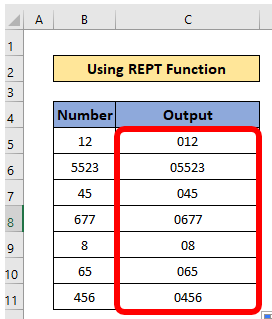2. The formula will add two zeros in front of all numbers, but it will not make numbers of the same digits.

If you want to make numbers of the same digits, you need to utilize the IF and LEN functions with the REPT function. Here, you will use the formula =IF((LEN(B5)<6),REPT(0,6-LEN(B5))&B5,B5), where;

LEN(B5) calculates the number of characters in cell B5

LEN(B5)<6) gives the condition that if the number of characters in cell B5 is less than 6.

REPT(0,6-LEN(B5))&B5 add the required zeros in front with cell B5.

3. Write the formula =IF((LEN(B5)<6),REPT(0,6-LEN(B5))&B5,B5) in cell C5.4. Copy and paste the formula into other cells or use the Fill Handle button.### The CONCAT (or CONCATENATE) Function

CONCAT or CONCATENATE function adds or merges characters of two cells, and can also add zeros in front of numbers in the cells. To use his formula:

1. Write =_xlfn.CONCAT("00",B5) in cell C5.2. Use the Fill Handle button to copy the formula into other cells.3. The formula will add two zeros to the cells, but will not give the cells the same digits.

### The RIGHT Function

Since the RIGHT function can take digits of a cell from the right, you can also use the formula to add zeros in front of numbers to make them specified digits. The formula’s syntax is =RIGHT(text, [num_chars]), where;

text represents what you will extract characters from the right.

num_chars is the number of characters you will extract from the right.

1. Write the formula =RIGHT("00"&B5,4) in cell C5.2. Use the Fill Handle icon to copy the formula to other cells.### Using the BASE Function

The BASE function converts numbers to another base, and is represented with the syntax =BASE (number, radix, [min_length]), where;

the number is the number you need to convert and must be an integer value (greater or equal to 0).

radix is the base radix to which you will convert the number (>=2 or <=36).

min_length is the minimum length of the string.

To use this formula, follow these steps:

1. Write the formula =BASE(B5,10,4) in cell C5.2. Copy the formula into other cells using the Fill Handle button.### Utilizing the Excel Power Query Tool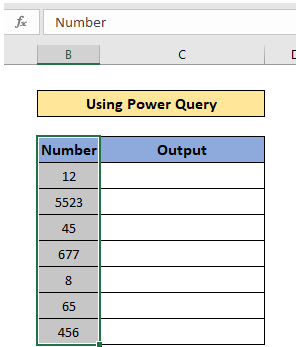Since the Excel Power Query Tool can transform data, you can also use it to add zeros in front of numbers following these steps:

1. Go to the Data tab, then Get Data, and Launch Power Query Editor.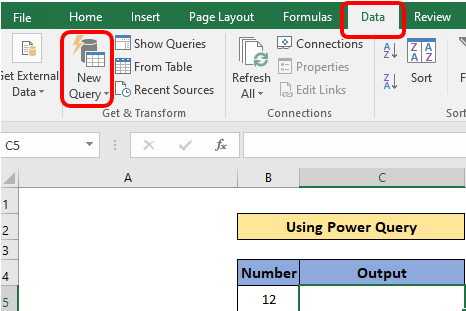2. When the Power Query Editor window opens, go to the Home tab, press the New Source drop-down menu, and click on Excel Workbook.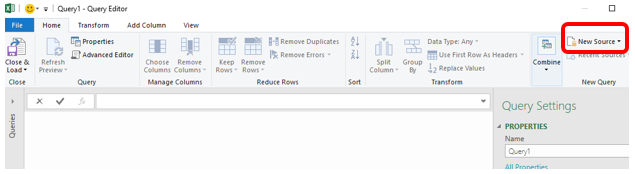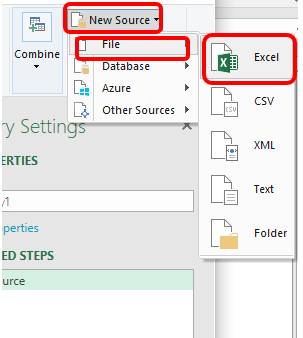3. Finally, extract data from the workbook.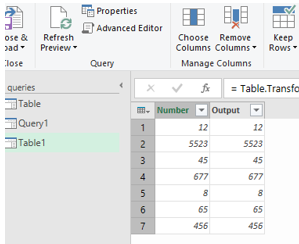4. You can also enter data manually by selecting the Enter Data option in the Power Query Editor.

5. After extracting data, you will need a new column to paste or input data manually and press OK.

6. Go to the Add Column tab and select the Custom Column option.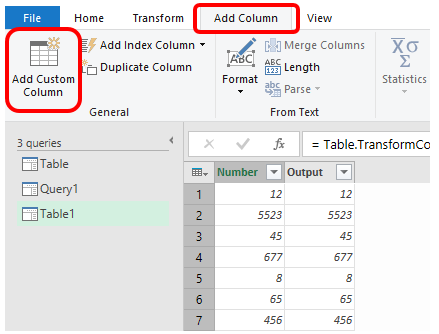7. When the Custom Column opens, give it your preferred name.

8. Paste the formula =Text.PadStart([Number],4,"0") and press OK.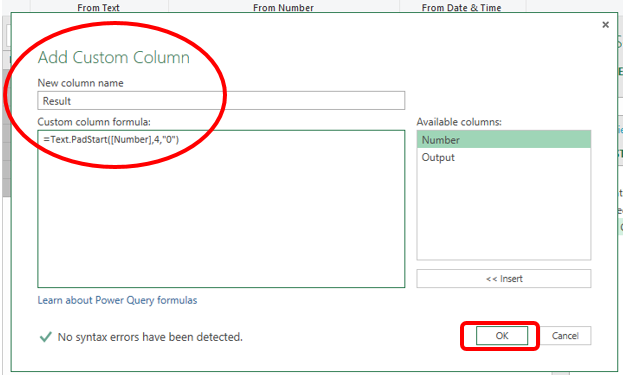9. A new column named Result will appear and will have cells of 4 digits with zeros in front.

10. Click on Close & Load, which is open a new sheet in the workbook with the data from the Power Query.